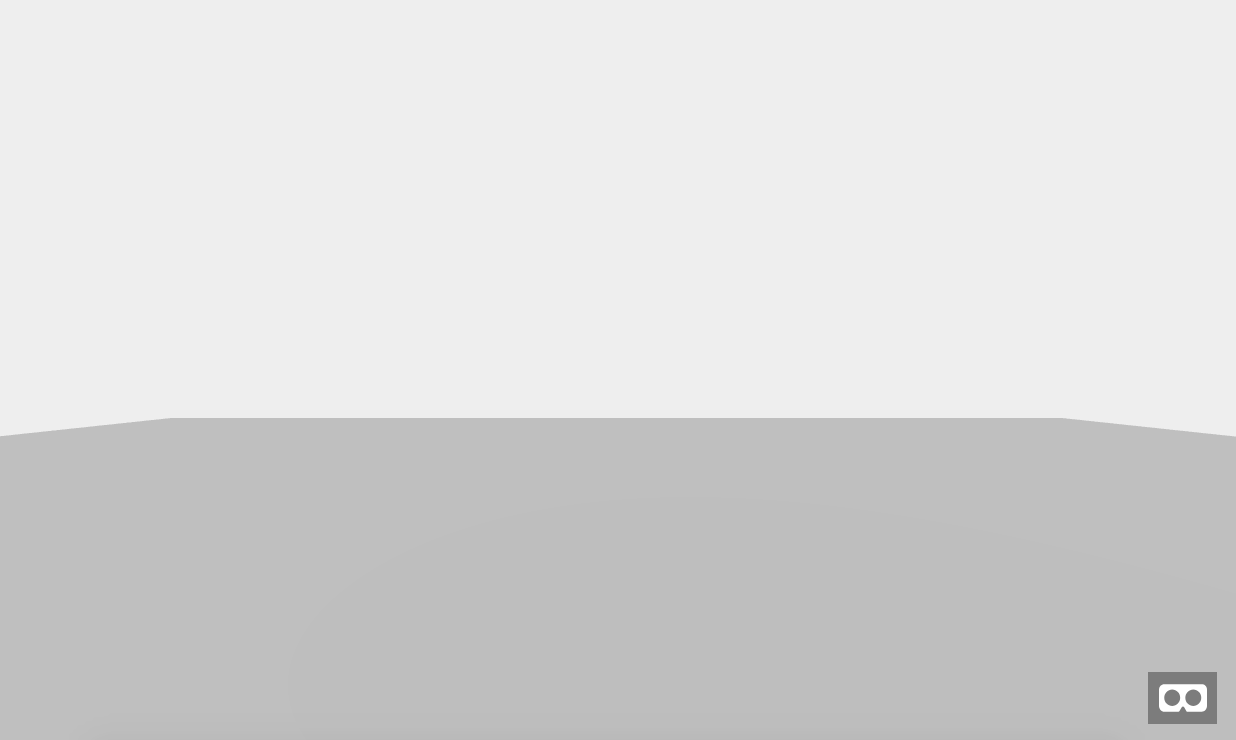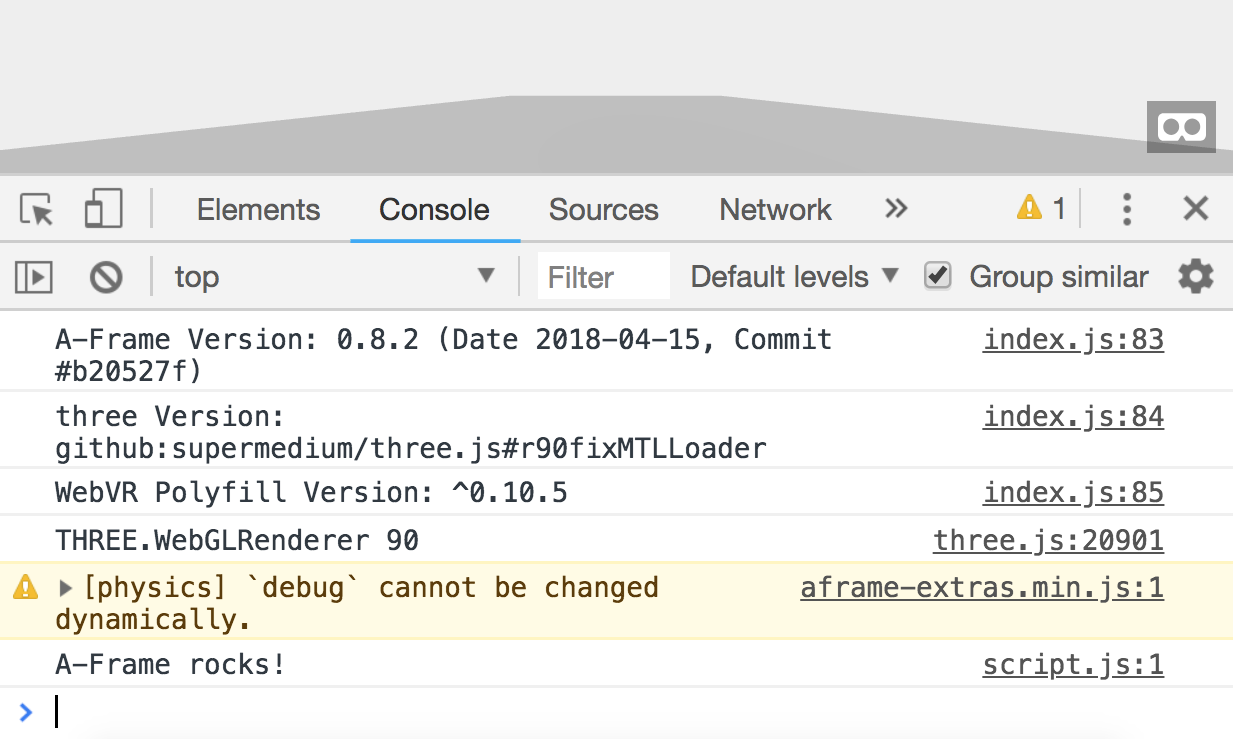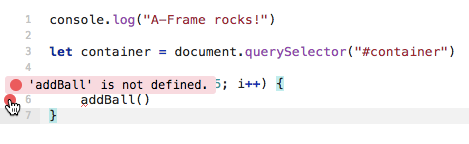# Setup

`<!DOCTYPE html><html>  <head>    <meta charset="utf-8">    <title>Hello, WebVR! • A-Frame</title>    <meta name="description" content="Hello, WebVR! • A-Frame">    <script src="https://aframe.io/releases/0.8.2/aframe.min.js"></script>  </head>  <body>    <a-scene background="color: #ECECEC">      <a-box position="-1 0.5 -3" rotation="0 45 0" color="#4CC3D9" shadow></a-box>      <a-sphere position="0 1.25 -5" radius="1.25" color="#EF2D5E" shadow></a-sphere>      <a-cylinder position="1 0.75 -3" radius="0.5" height="1.5" color="#FFC65D" shadow></a-cylinder>      <a-plane position="0 0 -4" rotation="-90 0 0" width="4" height="4" color="#7BC8A4" shadow></a-plane>    </a-scene>  </body></html>`
`<!DOCTYPE html><html>  <head>    <meta charset="utf-8">    <title>Hello, WebVR! • A-Frame</title>    <meta name="description" content="Hello, WebVR! • A-Frame">    <script src="https://aframe.io/releases/0.8.2/aframe.min.js"></script>  </head>  <body>    <a-scene>    </a-scene>  </body></html>`

`<script src="//cdn.rawgit.com/donmccurdy/aframe-extras/v3.13.1/dist/aframe-extras.min.js"></script>`

# Setting the scene

`<a-sky color="#eee"></a-sky>`
`<a-plane static-body position="0 0 0" rotation="-90 0 0" width="30" height="30" color="black" material="transparent: true; opacity: 0.2"></a-plane>`A light grey background with a darker grey floor
`<a-entity id="container"></a-entity>`

`<script src="script.js"></script>`
`console.log("A-Frame rocks!")`Check out the last message: “A-Frame rocks!”

# Adding balls dynamically

`let container = document.querySelector("#container")`
`for (let i = 0; i < 75; i++) {    addBall()}`Errors are just a normal part of a developer’s life.

# Writing a function to add a ball

`function addBall() {    let x = Math.random() * 10 - 5    let y = Math.random() * 50 + 2    let z = Math.random() * -10    container.innerHTML += `<a-sphere dynamic-body position="\${x} \${y} \${z}" radius="0.5" color="dodgerblue" mass="0.5"></a-sphere>`}`
• We can produce random numbers with the formula `Math.random() * MAX + MIN` (with MAX and MIN being numbers; MAX is more like a range if your MIN is greater than 0)
• I can add to the HTML inside the `container` element by accessing its `innerHTML`
• I can interpolate (insert) the variables `x`, `y`, and `z` into my string of HTML by surrounding the whole HTML string with backticks `` and surrounding each variable with a dollar sign and curly braces `\${}`

# Making the balls randomly colored

`let colors = ["red", "orange", "yellow", "green", "blue", "purple", "hotpink"]`
• `Math.random()` to produce a random number
• `colors.length` to get the length of the `colors` list (our MAX value)
• `Math.floor()` to ensure I get an integer (whole number)
`colors[Math.floor(Math.random() * colors.length)]`
`container.innerHTML += `<a-sphere dynamic-body position="\${x} \${y} \${z}" radius="0.5" color="\${colors[Math.floor(Math.random() * colors.length)]}" mass="0.5"></a-sphere>``